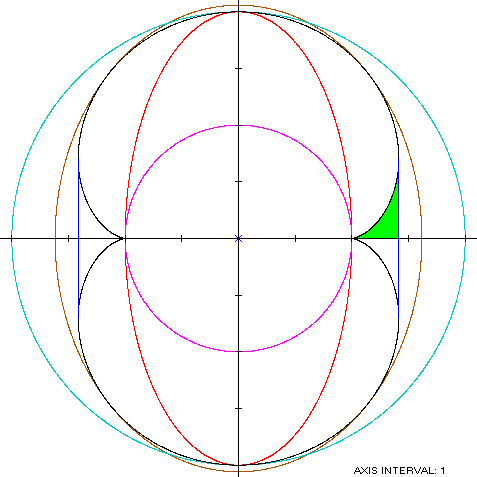Nephroid
NEPHROID

Balmoral SoftwareThe nephroid is a two-cusped epicycloid S with parametric equations
x(t) = 3cos(t) - cos(3t)

y(t) = 3sin(t) - sin(3t), 0 ≤ t < 2π

This bisymmetric curve is traced out in a counterclockwise direction around the origin, starting from the cusp at (2,0). Its maximum width occurs at the point pairs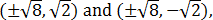which determine that the nephroid is non-convex by the multiple local extrema test. The values of t at these point pairs are ±π/4 (right pair) and ±3π/4 (left pair). The maximum height of the nephroid is between the point pair (0,±4), so the width x height of its bounding rectangle is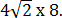### Metrics

The perimeter of the nephroid is 24 and its area A = 12π.

### Convex Hull

The convex hull is created by connecting the extreme abscissa points with vertical line segments of lengthas shown in blue in the left diagram below. We have
x'(t) = -3sin(t) + 3sin(3t)

y'(t) = 3cos(t) - 3cos(3t)

so by (L1), the perimeter of the convex hull iswhich is about 6% shorter than that of the nephroid.

To compute the area of the convex hull, we can see by (A1) that the area A1 of the region in green in the left diagram below is the integral of y dx from x = 2 at the cusp to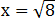at the maximum abscissa point:Therefore, the area of the convex hull is
A + 4A1 = 12π + 4(5 - 3π/2) = 20 + 6π = 38.849556,
which is about 3% more than the area of the nephroid.

### Boundary Circles & Inellipse

The squared-distance function of S
x2(t) + y2(t) = 10 - 6cos(2t)
has its extrema on the coordinate axes at (±2,0) and (0,±4), so the inradius is 2 and the circumradius is 4. A candidate for the inellipse is one enclosed by the annulus between these two circles, with a = 2 and b = 4. For verification, we have### Circumellipse

From Lemma B,
x(t)y(t) = [9sin(2t) - 6sin(4t) + sin(6t)]/2
is maximized in the first quadrant when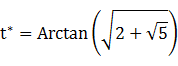The circumellipse dimensions are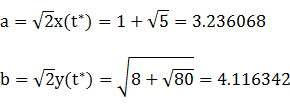For verification, we have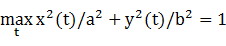### Summary Table

 Perimeter Area Centroid Figure Parameters Incircle R = 2 12.566371 12.566371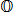Inellipse a = 2b = 4 19.376896 25.132741 Nephroid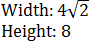24 37.699112 Convex hull 22.627417 38.849556 Circumellipse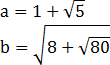23.181126 41.848410 Circumcircle R = 4 25.132741 50.265482